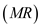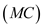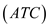# Quiz 9: Price Takers and the Competitive Process

False. Cost minimizing (or profit maximizing) condition requires equality between firm's marginal revenueequates its marginal cost. Given efficient production, if price in the market is greater than (or equal to) the average total cost, the operator will make positive economic (or normal) profit. Graph However, if the average total cost exceeds the price, the operator will incur economic loss. Graph Thus, whether an operator will make normal profit or not depends upon the position of average cost curve.